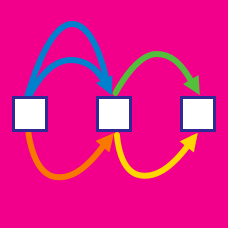Probability

Rule of Sum and Rule of Product: Level 2 Challenges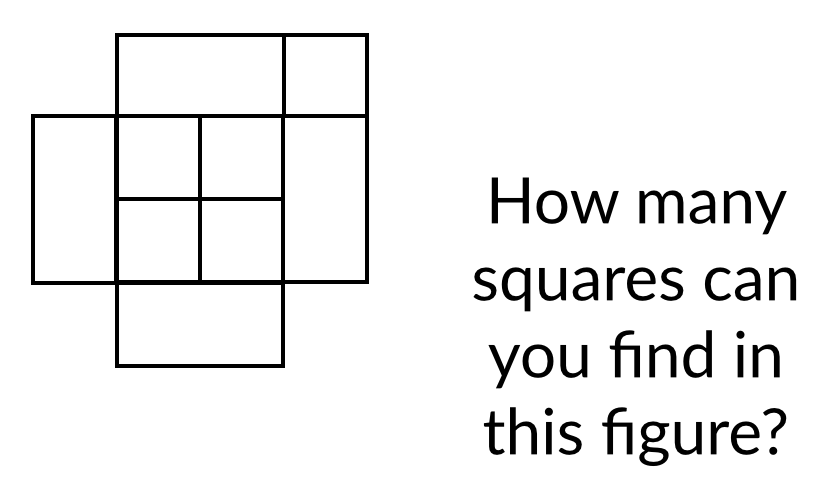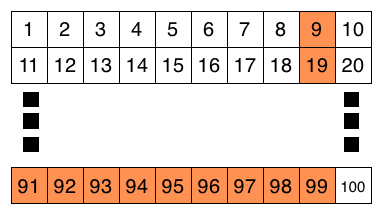When we write the integers from 1 to 100, how many times does the digit 9 appear?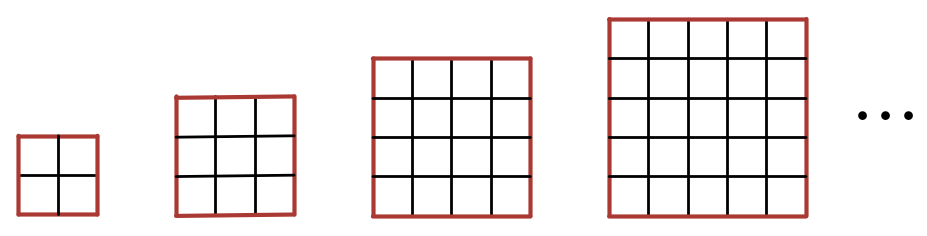Which of the following expresses the correct formula for the number of unit squares with exactly 1 red side in an $n \times n$ square with $n \geq 2$ ?

Find the number of $2$ digit numbers whose digit sum is a perfect square.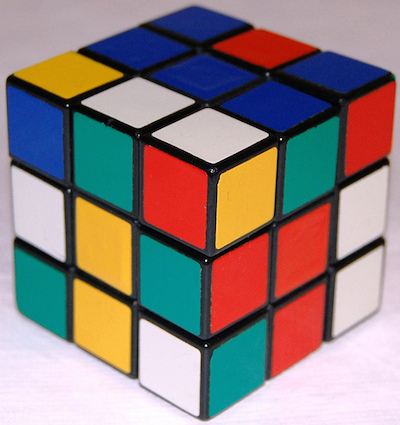If you take a $3 \times 3 \times 3$ Rubik cube and break it up into the $3^3$ cubes, how many cubes would have stickers on exactly $2$ faces?

Bonus question: Can you generalize this for a $N \times N \times N$ Rubik cube?

×